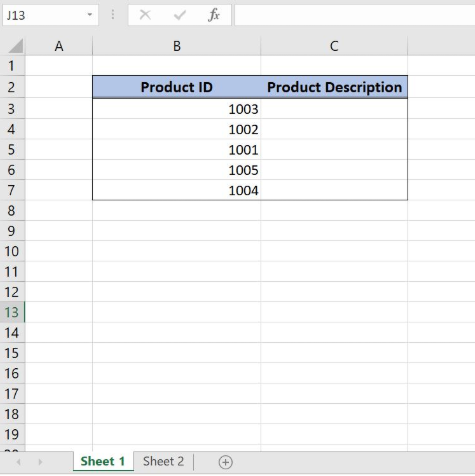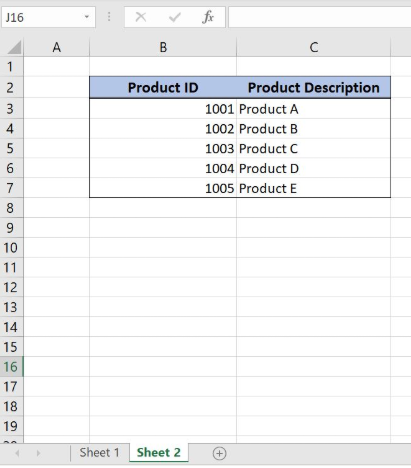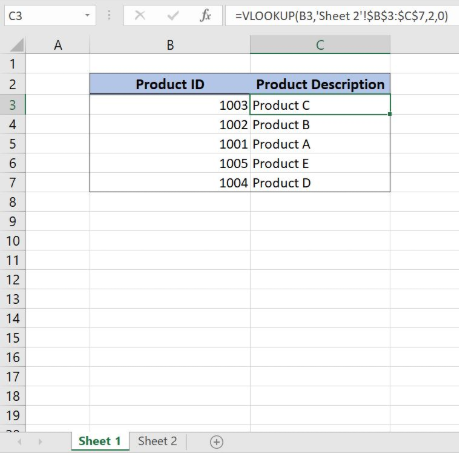Get instant live expert help with Excel or Google Sheets“My Excelchat expert helped me in less than 20 minutes, saving me what would have been 5 hours of work!”

#### Post your problem and you'll get expert help in seconds

Your message must be at least 40 characters
Our professional experts are available now. Your privacy is guaranteed.

# VLOOKUP from Another Sheet: Formula Examples

While working in Excel, we will often need to get values from another sheet. This is possible by using the VLOOKUP function. In this tutorial we will learn how to get values from another worksheet in Excel, using VLOOKUP function.

## Syntax of the VLOOKUP formula

The generic formula for getting values from another worksheet looks like:

`=VLOOKUP(lookup_value, ’sheet_name’!range, col_index_num, range_lookup)`

The parameters of the VLOOKUP function are:

• lookup_value – a value that we want to find in another worksheet
• ’sheet_name’!range – a range in another worksheet in which we want to lookup
• col_index_num – a column number in another worksheet from which we would like to pull a value
• range_lookup – default value 0. This means that we want to find an exact match for a lookup value.Figure 1. “Sheet 1” with the resulting tableFigure 2. “Sheet 2” from which we want to get data

We will now look at the example to explain in detail how this function works. Let’s start with examining the structure of the data that we will use.

In “Sheet 1” we have a table in which we want to pull data, while in “Sheet2” we have the table from which we want to pull data. Both tables consists of “Product ID” (column B) and “Product Description” (column C).

Get the Product description from Sheet 2 using VLOOKUP

Our goal is to obtain data from the “Product Description” column in the second worksheet and populate it into the same column of the 1st worksheet. This will be done based on each corresponding Product.Figure 3. “Sheet 1” with pulled data in “Product Description” column from “Sheet 2”

The formula looks like:

`=VLOOKUP(B3,'Sheet 2'!\$B\$3:\$C\$7,2,0)`

In our example, the lookup_value is the individual cell in “Product ID” column. The parameter ’sheet_name’!range is ‘Sheet 2’!\$B\$3:\$C\$7 because we want to find value from the range B3:B7 in “Sheet 2”. Col_index_num has value 2, as we want to pull value from the second column of the range. Finally, rang_lookup has value 0, because we want to find an exact match of “Product ID” values.

We must lock our lookup table when copying the formula to other cells (\$ before B3:C7 range).

To pull values from another worksheet, we need to follow these steps:

• Select cell C3 and click on it
• Insert the formula: `=VLOOKUP(B3,'Sheet 2'!\$B\$3:\$C\$7,2,0)`
• Press enter
• Drag the formula down to the other cells in the column by clicking and dragging the little “+” icon at the bottom-right of the cell.

As a result, we will get Product C in the cell B3. As you can see, the value of “Product ID” in B3 on the “Sheet 1” is 1003, while in the “Sheet 2” Product description for 1003 is Product C. The function pulls this value and returns it to “Sheet 1” as a result.

Most of the time, the problem you will need to solve will be more complex than a simple application of a formula or function. If you want to save hours of research and frustration, try our live Excelchat service! Our Excel Experts are available 24/7 to answer any Excel question you may have. We guarantee a connection within 30 seconds and a customized solution within 20 minutes.